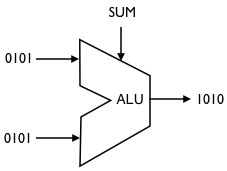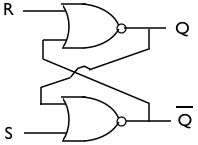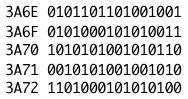# Weekly notes

In this class we will go over many concepts that will be new to you. We will try to not pull concepts magically out of a hat and instead explain where things come from; however, because our goal is only to build a computer, we will not go into depth into many of these concepts.

Most of these concepts are not difficult; they are simply ‘different’. As an example, binary arithmetic really is not difficult at all, particularly when compared with decimal arithmetic that you probably already mastered. However, it is ‘different’ so it will appear confusing for a while. In this page I will post handouts and reading suggestions that might help you understand the material better.

Most of the reading material will come either from ‘Code’ by Charles Petzold, or from ‘The elements of Computing Systems’ by Nisan and Schocken. ‘Code’ is not a textbook and is the most accessible of the two books; ‘The elements of Computing Systems’ is a textbook and will take us a few months to read if done properly, working out the exercises.

## Class intro

1_CD_I_class_description

Computer Design Handout

## 1. Circuits

• analog vs. digital electronics
• electric components: batteries, resistors, lamps, transistors, LED
• reading circuits and assembling circuits## 2. Combinatorial logic Design

• truth tables
• basic logic gates: not, and, or, nand, nor, xor
• multiplexers, demultiplexers

## 3. Binary Arithmetic

• binary representation
• the arithmetic logic unit (ALU)## 4. Sequential logic design

• timing
• data flip-flops
• registers, memory, counters
• RAM

• Code, ch. 14, “Feedback and Flip-flops”
• Code, ch. 16, “An assemblage of memory”## 5. Machine language

• the BASIC language and the GOTO logic
• arithmetic and logic operations
• memory access
• flow of control